In this blog, you'll learn about some of the common uses of diodes. Being the most basic semiconductor component, Diode has a wide range of electronic and electrical circuits applications to obtain desired results. Diodes are two-terminal semiconductor devices that conduct electrical current in only one direction when the potential difference across two terminals exceeds a certain limit. Their non-linear I-V characteristics make them suitable for various electronic and electrical applications.

Some Common Applications of Diodes are:

• Rectifiers
• Voltage Multipliers
• Clipper Circuit
• Clamping Circuit
• Protection Circuit
• In Logic Gates
• Flyback Circuits
• Light Emission
• Light Detection
• AM Envelope Detector
• Frequency Mixer

Diode as Rectifier

Rectifiers are used in AC to DC power supplies; The diodes were first used for rectification purposes to convert the AC signal into a unidirectional DC signal. A P-N junction diode allows electric current in forward bias conditions and blocks electric current in reverse bias conditions. Different types of rectifier circuits may be built using diodes. Half wave, center-tapped full-wave rectifier and full bridge rectifier circuits are the most common kinds. In most power conversion applications, a single or a combination of four diodes is utilized.

Half Wave Rectifier

Only one half cycle is allowed to pass in a half-wave rectifier, while the remaining half cycle is blocked. As a result, the half-wave rectifier wastes roughly half of the input power. Furthermore, a half-wave rectifier's output current or voltage is a pulsing DC rather than a pure DC.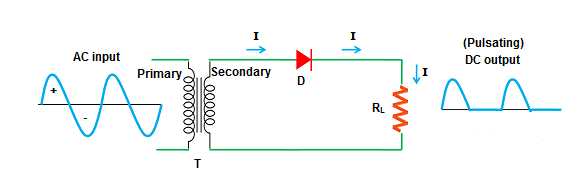Centre-Tapped Full Wave Rectifier

The key benefit of centre tapped full wave rectifier is that it allows electric current during the input AC signal's positive and negative half-cycles. As a result, the DC output of the centre-tapped full-wave rectifier has full-wave output in a single phase. The DC output of the centre-tapped full-wave rectifier is smoother than the half-wave rectifier. The diode's peak inverse voltage (PIV) in the centre-tapped full-wave rectifier is twice the transformer secondary terminal voltage that needs diodes with higher breakdown voltages.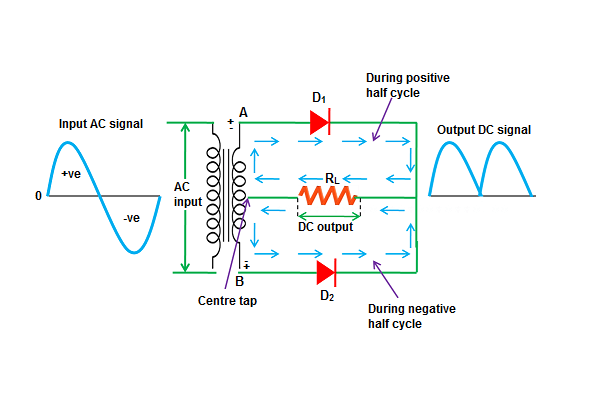Bridge Rectifier

A bridge rectifier is a full-wave rectifier that employs four or more diodes in a bridge circuit to effectively convert Alternating Current (AC) to Direct Current (DC). The Bridge rectifier's efficiency is nearly equal to that of a centre-tapped full-wave rectifier.
The transformer secondary voltage is equal to the diode's peak inverse voltage PIV. As a result, this type of rectifier is suitable for high-voltage applications. The transformer used in the Bridge rectifier circuit is smaller than that of the centre-tapped bridge rectifier.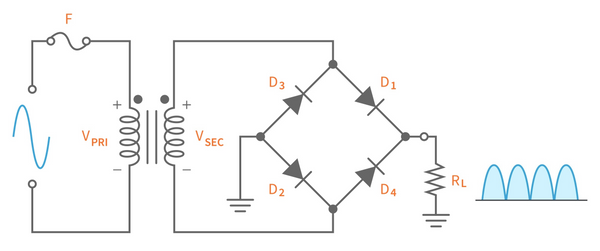Voltage Multipliers

Voltage multipliers and rectifiers are similar in many ways; they convert AC-to-DC voltages for use in many electrical and electronic circuit applications such as in microwave ovens, strong electric field coils for cathode-ray tubes, electrostatic and high voltage test equipments, etc. where a very high DC voltage is required from a relatively low AC supply.DC output voltage (Vdc) of a rectifier is usually restricted up to the peak value of its sinusoidal input voltage. However, by combining rectifier diodes and capacitors, we can effectively multiply this input peak voltage to produce a DC output equal to an odd or even multiple of the AC input voltage.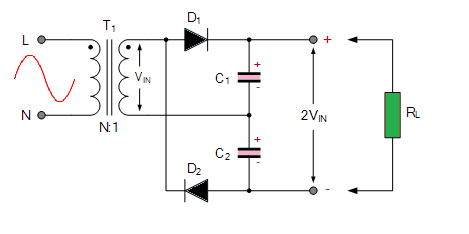A basic symmetrical voltage multiplier circuit made up of two half-wave rectifier circuits is shown above. We may enhance the output voltage of a normal half-wave rectifier by a fixed amount by adding a second diode and capacitor to its output. Because one of the diodes conducts in each half cycle, similar to a full wave rectifier circuit, this sort of voltage multiplier setup is known as a Full Wave Series Multiplier
Working

Capacitor C1 charges up through diode D1 when the sinusoidal input voltage is positive, while capacitor C2 charges up through diode D2 when the sinusoidal input voltage is negative. The output voltage 2VIN is measured across the two capacitors in series.

DC Voltage Doubler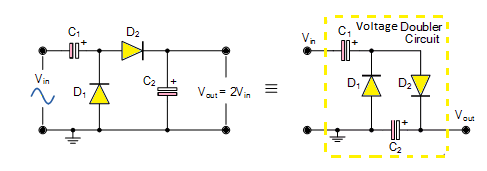So, how does it function? A half-wave voltage doubler is shown in the circuit. Diode D1 is forward biassed and conducts charging up the pump capacitor, C1, to the peak value of the input voltage during the negative half cycle of the sinusoidal input waveform (Vp). Due to the absence of a discharge path for capacitor C1, it remains fully charged and acts as a storage device in series with the voltage supply. Simultaneously, diode D2 conducts through D1, charging up capacitor C2.

Diode D1 is reverse biassed during the positive half cycle, preventing C1 from being discharged, while diode D2 is forward biassed, charging capacitor C2. Because there is a voltage across capacitor C1 already equals to the peak input voltage, capacitor C2 charges to twice the peak voltage value of the input signal. D1 charges C1 to Vp and on the positive half-cycle D2 adds the AC peak voltage to Vp onC1 and transfers it all to C2. Vout is the voltage across capacitor C2 discharges through the load is equals to 2Vp.

Diode in Clipper Circuit

A clipper is a diode-based circuit that is used to reshape the waveform of a signal by clipping or cutting a piece of the positive, negative, or both portions of the signal. It is used to set a voltage restriction at a certain point without affecting the rest of the input waveform. Clipping Circuits are used in FM transmitters to confine noise peaks to a specific amount, removing excessive peaks from the signal.

 Series Shunt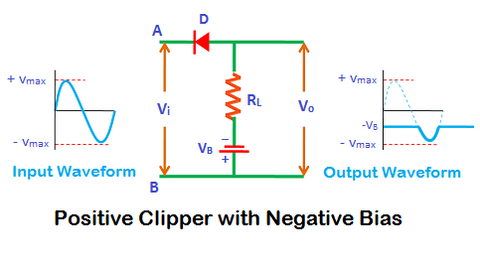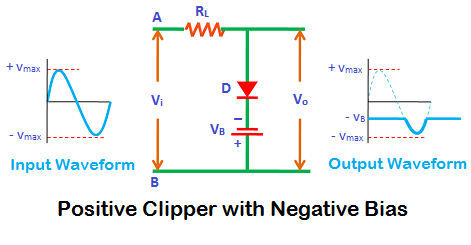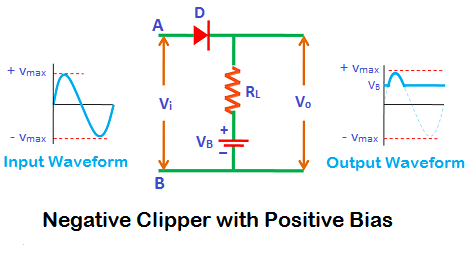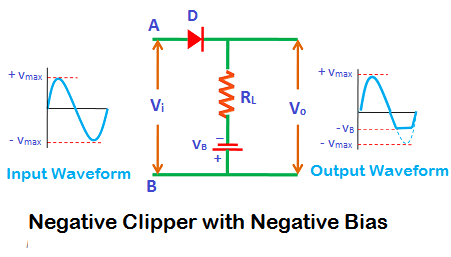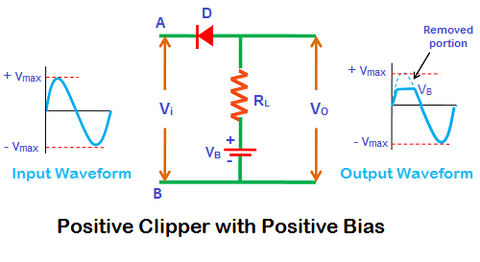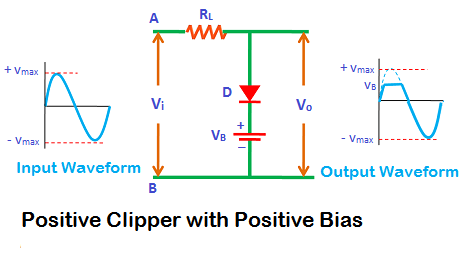Next>>

Active components